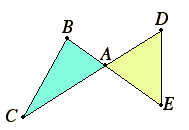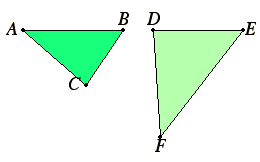# Definition 2

Two figures are reciprocally related when the sides about corresponding angles are reciprocally proportional.

## Guide

 This isn’t the actual definition that appears, but an approximation of its intent. A literal translation is incomplete, and this definition may have been added after Euclid. The intention can be seen in proposition VI.15 as illustrated here.The proposition states that if two triangles have one angle equal to one angle, then the triangles are equal if and only if the sides about the equal angles are reciprocally proportional. In the figure BAC and DAE are equal angles. So the two triangles are equal if and only if CA : AD = EA : AB. Euclid doesn’t define the term “reciprocally proportional,” but the meaning of the term is clear from its use.Although Euclid doesn’t address the question, it would be interesting to characterize which triangles are reciprocally related, as shown to the left. The conditions are that AB : DE = DF : AC, BC:EF = DE : AB, and AC : DF = DF : BC. Multiplicatively, AB DE = BC EF = AC DF.